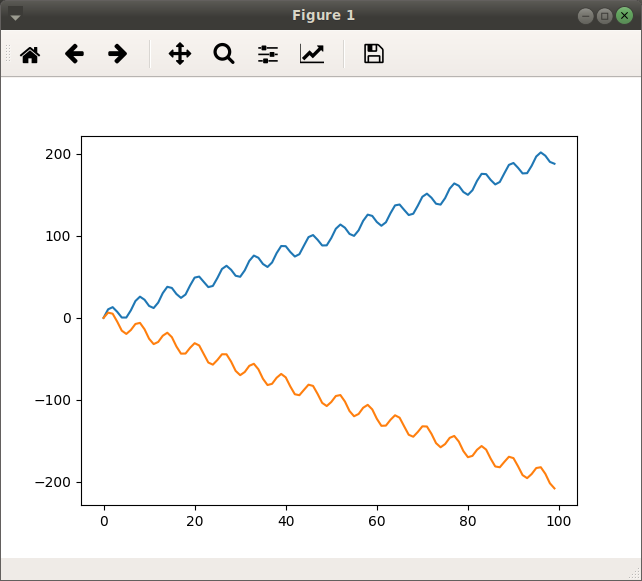Skip to content

# keras-team/keras

Have a question about this project? Sign up for a free GitHub account to open an issue and contact its maintainers and the community.

By clicking “Sign up for GitHub”, you agree to our terms of service and privacy statement. We’ll occasionally send you account related emails.

Already on GitHub? Sign in to your account

# Can keras handle sequence-specific parameters? #12654

Open
opened this issue Apr 10, 2019 · 2 comments

## Comments

Projects
None yet

### cranedroesch commented Apr 10, 2019

 I am not sure if this is a feature request or an implementation question. It's the former if what I want to do is not possible, and the latter if it is. Anyway, it is a cross-post with a SO thread, but I'm not getting any bites over there, which makes me suspect that it isn't currently possible. Consider the following data: `````` t = 100 x = np.array(list(range(t))).reshape(1, t) B = np.array([2,-2]).reshape(1,2) y = x.T @ B + 10*np.vstack([np.sin(x), np.sin(x)]).T x = x y = y.T plt.clf() plt.plot(x, y[0,:]) plt.plot(x, y[1,:]) ``````I want to fit a semiparametric model, where a LSTM learns the sine wave, and a linear regression learns the trend for each time series. `````` y = x * b + LSTM(x) (N, t, 1) (t,p)(p,N) (N, t, 1) `````` Transposing the first term, we've got: `````` y = (x * b).T + LSTM(x) (N, t, 1) (N,t) (N, t, 1) `````` How can I implement this in Keras? Is it possible? I'm running into problems because `x` has a fixed dimension -- it's not something that you'd take minibatches of. Likewise, the weight matrix `b` has a fixed size. `N` is constant in my problem -- there are a fixed number of sequences in the world, and there will never be more. If I were doing this by hand, there would be a very obvious form to the gradients. But it's just not clear how to shoe-horn this into something Keras can work with. Here's a crack at coding it up: `````` y = y.reshape(2,t,1) x = x.reshape(1,t,1) Linp = Input(shape = (100,1)) xinp = Input(shape = (100,1)) lstm = LSTM(1, return_sequences = True)(Linp) XB = Dense(2, use_bias = False)(xinp) rs = Lambda(lambda x: K.reshape(x, (2,100,1)))(XB) added = add([rs, lstm]) m = Model([xinp, Linp], added) m.summary() m.compile(optimizer = "Adam", loss = "mean_squared_error") m.fit([x,np.vstack([x,x])], y) `````` It fails with the error ` ValueError: All input arrays (x) should have the same number of samples. Got array shapes: [(1, 100, 1), (2, 100, 1)]`
Contributor

### briannemsick commented Apr 12, 2019 • edited

 Generally speaking, it is most common to fit and remove the linear component (can be swapped out for other model types) using your favorite other software package (sklearn, ...) and fit the deep learning model on the residual (y - x*b in your notation). For this specific model you could construct a graph that does (x->lstm blocks, x-> dense, add dense + lstm block, loss on y).
Author

### cranedroesch commented Apr 12, 2019 • edited

 @briannemsick Thanks for the reply. I could certainly do this, but it'd ignore correlations between the terms being modeled parametrically and the terms being modeled nonparametrically. The reason that you don't want to do that is that the gradients of either component will not be invariant to the values of the other, as the residuals will be continuously updated during the fitting process. I don't follow your second paragraph exactly. Are you proposing a method to execute the partitioned model, or a method to jointly estimate the parametric and nonparametric components? And, in case it doesn't go without saying, my real use-case isn't the toy model that I put in the example.
to join this conversation on GitHub. Already have an account? Sign in to comment
You can’t perform that action at this time.